UPSC  >  Solved Examples: Time & Distance

# Solved Examples: Time & Distance - Notes | Study UPSC Prelims Paper 2 CSAT - Quant, Verbal & Decision Making - UPSC

 1 Crore+ students have signed up on EduRev. Have you?

The Basics of Time, Speed & Distance
Funda 1: Average Speed We know that the average speed in a journey is given by (Total Distance Covered) / (Total Time Taken); but there are few special cases which might help in solving questions –
If the distance covered is constant (in each part of the journey, then the average speed is Harmonic Mean of the values.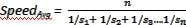If the time taken is constant (in each part of the journey then the average speed is Arithmetic Mean of the values.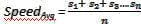Funda 2: Using Progressions (Arithmetic & Harmonic) In many questions, you come across a situation when a person is going from Point A to Point B at various speeds and taking various times. We know that if distance is constant, speed and time are inversely proportional to each other. But, this information can also be used to deduce these two facts –

• If the various speeds which are mentioned are in AP, then the corresponding times taken will be in HP.
• If the various speeds which are mentioned are in HP, then the corresponding times taken will be in AP.

Let us use these ideas to solve couple of quant questions.

Eg 2.1 Arun, Barun and Kiranmala start from the same place and travel in the same direction at speeds of 30,40 and 60 km per hour respectively. Barun starts two hours after Arun. If Barun and Kiranmala overtake Arun at the same instant, how many hours after Arun did Kiranmala start? [CAT 2006]
Note: Arun Barun Kiranmala is a 1968 Bangladeshi film. Now you can guess what inspires CAT question setters.
Solution: As you can see that the speeds are in HP, so we can say that the times taken will be in AP. Time difference between Arun and Barun is 2 hours, so the time difference between Barun and Kiranbala will also be 2 hours. Hence, Kiranbala started 4 hours after Arun.

Eg 2.2 Rishi Kapoor can swim a certain course against the river flow in 84 minutes; he can swim the same course with the river flow in 9 minutes less than he can swim in still water. How long would he take to swim the course with the river flow?
Solution: Let us say Speed of the Rishi Kapoor in still water is ‘RK’ and Speed of the river is ‘R’. Hence, Rishi Kapoor’s speeds against the river flow, in still water and with the river flow are: RK – R, RK and RK + R. As you can see, they are in AP. Hence, the corresponding times taken will be in HP. Let us say that the time taken to row down with the stream is ‘t’, then 84, t+9 and t are in HP. So,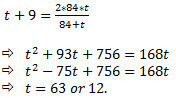Funda 3: Special Case Let us say that two bodies ‘a’ & ‘b’ start at the same time from two points P & Q towards each other and meet at a point R in between. After meeting at R, ‘a’ takes ta time to reach its destination (Q) and ‘b’ takes tb time to reach its destination (P); then.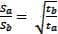Also, the time taken by ‘a’ & ‘b’ to meet (i.e. to reach point R from P & Q respectively) is given by: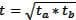Note: The same formulae will be valid if two bodies ‘a’ & ‘b’ start at different times from two points P & Q towards each other. They meet at a point R in between after travelling for ta and tb time respectively. After meeting, they take the same amount of time (‘t’) to reach their respective destinations (Q & P).

Time and Distance - Examples (with Solutions)
1. The fundamental relationship between Distance (s), time (t) and speed (v) is given by: s = v x t.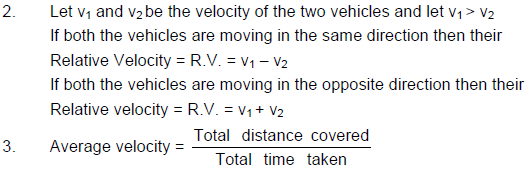If x& x2 are the distances covered at velocities v1 & v2 respectively then the average velocity over the
entire distance (x1 + x2) is given by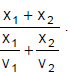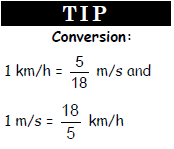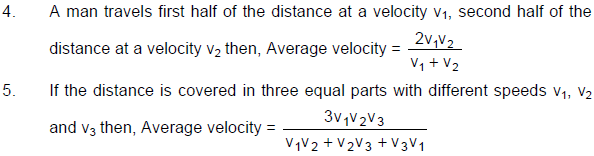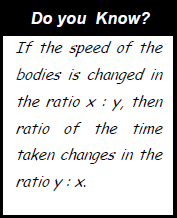6. For the same distance, the time is inversely proportion to the speed of the
object. These types of problems can be solved as the problems of
percentage.

Ex.1 If I decrease my speed by 20% of original speed, I reach office 7 minute late. What is my usual time and new time of reaching office?
Sol. Since speed is decreased by 20% i.e.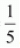of the original. New speed will become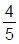of the original
speed. For the same distance, the time will become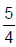of original time. Therefore new time increase by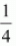of the original. This is given equal to 7 minutes.
So Usual time = 7 × 4 = 28 minutes and New time = 28 + 7 = 35 minutes.

7. When time is constant the ratio of speeds of the object is equal to the ratio of the distance covered by
them i.e.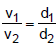Ex.2 A train leaves Calcutta for Mumbai, a distance of 1600 kms at the same time a train leaves Mumbai to Calcutta. The trains meet at Nagpur which is at a distance of 700 kms from Mumbai. What is the ratio of the speeds of the trains?
Sol. From the problem, it is clear that when the first train travels a distance of 1600 - 700 = 900 km, the
second train travels a distance equal to 900. So, the ratio of their speeds is 9 : 7.

Trains

(i) When a train approaches a stationary object (a tree, a stationary man, a lamp-post; we assume the
length of the object to be infinitely small; provided its length isn’t mentioned)
Time taken by the train to cross Pole =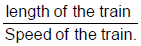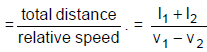Speed of the train.
length of the train

(ii) However, when a train approaches a platform, the time taken by the train to cross the platform is same
as the time taken by the train to cross a distance equal to its own length plus the length of the platform
at its own velocity.
∴Time taken to cross the platform =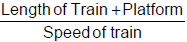(iii) For two trains having lengths l1 & l2 and traveling in the same direction with speeds v1 & v2 respectively
(v1 > v2).
Time taken to cross each other completely(iv) Similarly, for two trains traveling in the opposite direction:
Time taken to cross each other completely = total distance /relative velocity =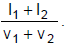(v) If two trains/Object start at the same time from two points X & Y and move towards each other and after
crossing they take a & b hrs respectively to reach opposite points Y and X, then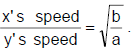Ex.3 A train 110 m long travels at 60 kmph. How long does it take?
(a) To pass a telegraph post by the side of the track?
(b) To pass a man running at 6 kmph in the same direction as the train?
(c) To pass a man running at 6 kmph in the opposite direction?
(d) To pass a station platform 240 m long?
(e) To pass another train 170 m long, running at 40 kmph in the same direction?
(f) To pass another train 170 m long, running at 60 kmph in the opposite direction?

Sol. (a) Speed of train =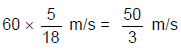∴ Time taken to cross the telegraph post =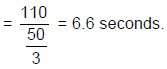(b) Speed of man =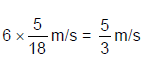∴ Time taken to pass the man = Length of the train/ Relative velocity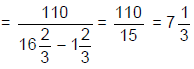seconds.

(c) Time = Length of the train/ Relative velocity =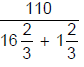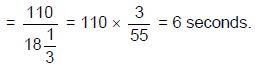(d) Time = (Length of the train + Length of platform) / Relative velocity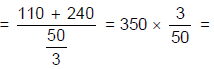21 seconds.

(e) Speed of the second train =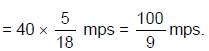∴ Time = Sum of the length of the two trains/ Relative velocity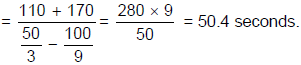(f) Time = Sum of the length of the two trains/Relative velocity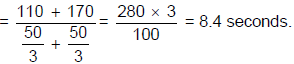Boats & Streams
Let Speed of boat in still water = b km/hr
Speed of stream = w km/hr
Speed of boat with stream (Down Stream), D = b + w
Speed of boat against stream (Up stream), U = b - w
Speed of boat in still water,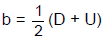Speed of stream,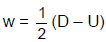Ex.4 A man can row 4.5 km/hr in still water. It takes him twice as long to row upstream as to row downstream. What is the rate of the current?
Sol. Speed of boat in still water (b) = 4.5 km/hr.
It is given upstream time is twice to that of down stream.
⇒ Downstream speed is twice to that of upstream.
So b + u = 2(b - u)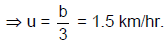Circular Motion
Circular Motion with two people

Ex.5 Sachin and Saurav, as a warm-up exercise, are jogging on a circular track. Saurav is a better athlete and jogs at 18km/hr while Sachin jogs at 9 km/hr. The circumference of the track is 500m (i.e. ½ km). They start from the same point at the same time and in the same direction. When will they be together again for the first time?
Sol. Method 1: Since Saurav is faster than Sachin, he will take a lead and
as they keep running, the gap between them will keep widening. Unlike
on a straight track, they would meet again even if Saurav is faster than
Sachin.

The same problem could be rephrased as “In what time would Saurav
take a lead of 500 m over Sachin”?

Every second Saurav is taking a lead of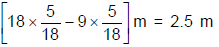over Sachin in Therefore, they would meet for the first time after 200
sec.

In general, the first meeting if both are moving in the same direction and
after both have started simultaneously occurs after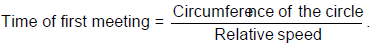Method 2: For every round that Sachin makes, Saurav would have made 2 rounds because the ratio of
their speeds is 1 : 2. Hence, when Sachin has made 1 full round, Saurav would have taken a lead of 1
round. Therefore, they would meet after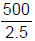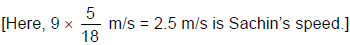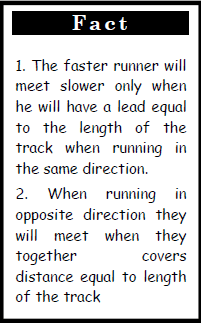Ex.6 Suppose in the earlier problem when would the two meet for the first time if they are moving in the opposite directions?
Sol. If the two are moving in the opposite directions, then
Relative speed = 2.5 + 5 = 7.5 m/s.
[Hence, time for the first meeting = Circumference / Relative speed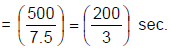Ex.7 If the speeds of Saurav and Sachin were 8 km/hr and 5 km/hr, then after what time will the two meet for the first time at the starting point if they start simultaneously?

Sol. Let us first calculate the time Saurav and Sachin take to make one full circle.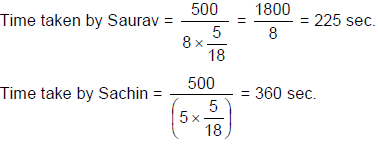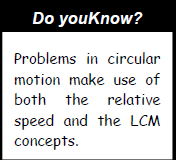Hence, after every 225 sec, Saurav would be at the starting point and after every 360 sec Sachin would
be at starting point. The time when they will be together again at the starting point simultaneously for
the first time, would be the smallest multiple of both 225 and 360 which is the LCM of 225 and 360.

Hence, they would both be together at the starting point for the first time after LCM (225, 360) = 1800
sec = 0.5 hr. Thus, every half an hour, they would meet at the starting point.

Note: From the solution you could realise that it is immaterial whether they move in the same direction
or in the opposite.

Circular motion with three people:
If three persons A, B, and C are running along a circular track of length d meters with speeds
Va ,Vb ,Vc respectively. To find the time when all the three will meet for the first time, we have to
calculate the relative time of the meeting of any one (A or B or C) among the three with other two
runners and then calculate the LCM of these two timings. This will be the time when all the three
runners will meet for the first time.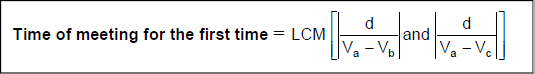And to calculate when they all meet for the first time at the starting point, we have to take the LCM of
the timings taken by all the runners separately to cover one full circular motion.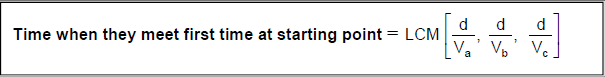Ex.8 Let us now discuss the cases of circular motion with three people: Laxman joins Saurav and Sachin, and all of them run in the same direction from the same point simultaneously in a track of length 500 m. Laxman moves at 3 km/hr, Sachin at 5 km/hr and Saurav at 8 km/hr. When will all of them be together again?
a. for the first time?
b. for the first time at the starting point?
Sol. (a) Break the problem into two separate cases.
In the first case, Saurav moves at the relative speed of (8 - 5) = 3 km/hr with respect to Sachin.
At a relative speed of 3 km/hr, he would meet Sachin after every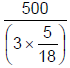= 600 sec = 10 min.

In the second case, Saurav moves at the speed of (8 - 3) km/hr = 5 km/hr with respect to Laxman.
At a relative speed of 5 km/hr, he would meet Laxman after every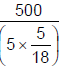= 360 sec = 6 min.

∴ If all the three have to meet, they would meet after every [LCM (10, 6)] min = 30 min or ½ hour.
Hence, they would all meet for the first time after 30 min.

(b) If we need to find the time after which all of them would be at the starting point simultaneously for the
first time, we shall use the same method as in the case involving two people.
At a speed of 8 km/hr, Saurav takes 225 sec. to complete one circle.
At a speed of 5 km/hr, Sachin takes 360 sec. to complete one circle.
At a speed of 3 km/hr, Laxman would take 600 sec. to complete one circle.
Hence, they would meet for the first time at the starting point after
LCM (225, 360, 600) sec. = 1800 sec.

Ex.9 A thief is spotted by a policeman from a distance of 200 m. When the policeman starts a chase, the thief starts running. Speed of thief is 10 Kmph and that of policeman is 12 kmph. After how many hours will the policeman catch the thief?
Sol.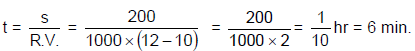Ex.10 A man steals a car at 1 : 30 pm & drives at 40 kmph. At 2 pm the owner starts chasing his car at 50 kmph. At what time does he catch the man?
Sol. Distance covered by the thief in 1h = 40 km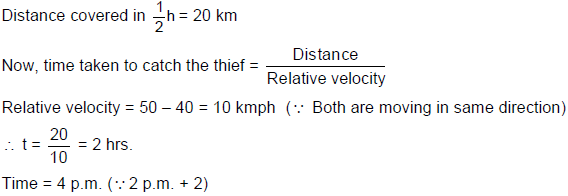The document Solved Examples: Time & Distance - Notes | Study UPSC Prelims Paper 2 CSAT - Quant, Verbal & Decision Making - UPSC is a part of the UPSC Course UPSC Prelims Paper 2 CSAT - Quant, Verbal & Decision Making.
All you need of UPSC at this link: UPSC

## UPSC Prelims Paper 2 CSAT - Quant, Verbal & Decision Making

67 videos|50 docs|151 tests

## UPSC Prelims Paper 2 CSAT - Quant, Verbal & Decision Making

67 videos|50 docs|151 tests

### How to Prepare for UPSC

Read our guide to prepare for UPSC which is created by Toppers & the best Teachers

Track your progress, build streaks, highlight & save important lessons and more!

,

,

,

,

,

,

,

,

,

,

,

,

,

,

,

,

,

,

,

,

,

,

,

,

;